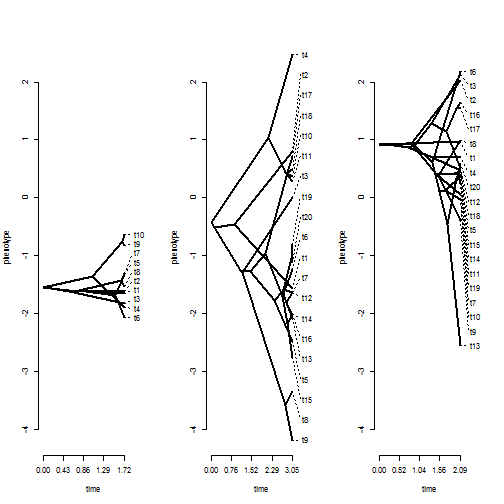## Sunday, March 19, 2017

### Simulation method for testing if the rate of phenotypic evolution differs among trees using `phytools::ratebytree`

As noted in some previous posts (e.g., 1, 2 3) I have written a function, `ratebytree`, to compare the rate of trait evolution under Brownian motion between different trees.

As I have previously pointed out this is essentially the censored rate test of described in an article by Brian O'Meara & colleagues from 2006.

I've been working on this as part of a project that is being conducted with members of Andrew Crawford's lab here at la Universidad de los Andes.

Prior versions of the method used a χ2 distribution to obtain a P-value for the comparison of one- and multiple rate models. A limitation of this approach is that though the theory of likelihoods tells as the (two-times-the) likelihood ratio should approach a χ2 distribution with degrees of freedom equal to the difference in parameterization of the two models, this property is asymptotic - meaning that for phylogenies that are relatively modest in size, we may not be able to realistically assume the likelihood-ratio to have a χ2 distribution under the null.

One relatively straightforward way to circumvent this issue, and one that is already implemented for other phytools function (such as the closely related function `brownie.lite`) is to use numerical simulation under the fitted null model to generate a null-distribution of the likelihood-ratio.

I have now implemented this method for `ratebytree`. The update can be obtained by installing phytools from GitHub.

Here's a quick demo:

``````library(phytools)
packageVersion("phytools")
``````
``````##  '0.5.84'
``````
``````## here is our trees & data:
trees
``````
``````## 3 phylogenetic trees
``````
``````x
``````
``````## []
##         t1         t2         t7         t8         t5         t6
## -1.6596753 -1.6202389 -1.3149799 -1.5414064 -1.3301366 -2.0684157
##         t3         t9        t10         t4
## -1.8276196 -0.8308954 -0.6392167 -1.8945305
##
## []
##          t4         t10         t11          t5         t13         t14
##  2.48011289  0.36885303  0.28941190 -2.48173563 -2.08700280 -2.00920263
##         t12         t19         t20          t7         t17         t18
## -1.63965832 -0.79959572 -1.12513496 -1.63145651  0.71196801  0.48481364
##          t6         t15         t16          t3          t8          t9
## -1.23720659 -2.75963479 -2.01630598  0.01276553 -3.34797694 -4.18915934
##          t1          t2
## -1.56362471  0.80736136
##
## []
##          t1          t8          t9         t14         t15          t7
##  0.96676584  0.99311776 -0.39647851  0.38995149  0.45217977  0.05880620
##          t2         t19         t20         t18         t13         t10
##  2.03242066  0.25253907  0.59278690  0.49438127 -2.55757363 -0.03192012
##         t11          t6          t3         t16         t17         t12
##  0.36683176  2.18782243  2.13977282  1.65146078  1.56860812  0.50089411
##          t4          t5
##  0.72104497  0.47858242
``````
``````## plotted:
ylim<-range(x)
par(mfrow=c(1,3))
````````````## now let's fit our models:
fit.chisq<-ratebytree(trees,x)
fit.chisq
``````
``````## ML common-rate model:
##  s^2  a   a    a    k   logL
## value    1.2177  -1.5456 -0.4248 0.9155  4   -69.8154
##
## ML multi-rate model:
##   s^2 s^2  s^2   a   a    a    k   logL
## value    0.337   1.3276  1.5482  -1.5456 -0.4248 0.9155  6   -66.6573
##
## Likelihood ratio: 6.3163
## P-value (based on X^2): 0.0425
##
## R thinks it has found the ML solution.
``````
``````fit.sim<-ratebytree(trees,x,test="simulation")
``````
``````## Generating null distribution via simulation -> |...........|
## Done!
``````
``````fit.sim
``````
``````## ML common-rate model:
##  s^2  a   a    a    k   logL
## value    1.2177  -1.5456 -0.4248 0.9155  4   -69.8154
##
## ML multi-rate model:
##   s^2 s^2  s^2   a   a    a    k   logL
## value    0.337   1.3276  1.5482  -1.5456 -0.4248 0.9155  6   -66.6573
##
## Likelihood ratio: 6.3163
## P-value (based on simulation): 0.099
##
## R thinks it has found the ML solution.
``````

For reference, the data for this demo were simulated as follows:

``````t1<-pbtree(n=10)
t2<-pbtree(n=20)
t3<-pbtree(n=20)
x<-fastBM(t1,sig2=1)
y<-fastBM(t2,sig2=2)
z<-fastBM(t3,sig2=2)
trees<-c(t1,t2,t3)
x<-list(x,y,z)
``````

In a subsequent post I will employ simulation to examine whether using the χ2 test actually results in elevated type I error (as we might expect in theory for small trees) compared to the practice of obtaining a null-distribution via simulation, as we have used above.